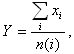# How are average exchange rates calculated?

In addition to daily foreign exchange market rates, the Czech National Bank publishes average exchange rates for individual months, cumulative months, quarters and years. The published figure is the arithmetic mean of the daily rates declared for the given period. The average exchange rate for the given period is published simultaneously with the last daily rate falling within that period.

Mathematical expression:Y......resulting average rate for the given period;
i......exchange rate declared for working day i;
n(i)....number of working days i in the given period.

If not all the daily rates are available for the given period (i.e. if an exchange rate was not given in the CNB exchange rate chart in a particular period), the average exchange rate is not calculated and no entry is made in the relevant cell of the table.av